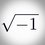A Hard Function Problem

Based on 2013 Olympiad Problem

A function $f$ is defined for all $x$ and has the following property,

$\large f(x) = \frac{ax+b}{cx+d}$

If $a, b, c$ and $d$ are real number and the function above satisfy $f(19)=19, f(97) = 97, f(f(x)) = x,$ for every $x$ value, except $\large -\frac{d}{c}$, find range of the functionNote by Jason Chrysoprase
3 years, 4 months ago

This discussion board is a place to discuss our Daily Challenges and the math and science related to those challenges. Explanations are more than just a solution — they should explain the steps and thinking strategies that you used to obtain the solution. Comments should further the discussion of math and science.

When posting on Brilliant:

• Use the emojis to react to an explanation, whether you're congratulating a job well done , or just really confused .
• Ask specific questions about the challenge or the steps in somebody's explanation. Well-posed questions can add a lot to the discussion, but posting "I don't understand!" doesn't help anyone.
• Try to contribute something new to the discussion, whether it is an extension, generalization or other idea related to the challenge.
• Stay on topic — we're all here to learn more about math and science, not to hear about your favorite get-rich-quick scheme or current world events.

MarkdownAppears as
*italics* or _italics_ italics
**bold** or __bold__ bold

- bulleted
- list

• bulleted
• list

1. numbered
2. list

1. numbered
2. list
Note: you must add a full line of space before and after lists for them to show up correctly
paragraph 1

paragraph 2

paragraph 1

paragraph 2

> This is a quote
This is a quote
# I indented these lines
# 4 spaces, and now they show
# up as a code block.

print "hello world"
# I indented these lines
# 4 spaces, and now they show
# up as a code block.

print "hello world"
MathAppears as
Remember to wrap math in $$...$$ or $...$ to ensure proper formatting.
2 \times 3 $2 \times 3$
2^{34} $2^{34}$
a_{i-1} $a_{i-1}$
\frac{2}{3} $\frac{2}{3}$
\sqrt{2} $\sqrt{2}$
\sum_{i=1}^3 $\sum_{i=1}^3$
\sin \theta $\sin \theta$
\boxed{123} $\boxed{123}$

Sort by:

Staff - 3 years, 4 months ago

Wow, that guy really wrote the same problem. We both came from Indonesia :)

- 3 years, 4 months ago

Sir, Can you please suggest me where can I learn about functions & solving these type of questions? Honestly, I don't know much about this topic , please help me, thank you.!

- 3 years, 4 months ago

Practice the challenge quizzes in the chapter and read the wikis.

Staff - 3 years, 4 months ago

Thank you sir :-)

- 3 years, 4 months ago

I'll assume that the domain is $\mathbb{R} - \{-\frac{d}{c} \}$

If $\dfrac{b}{a} \neq \dfrac{d}{c}$, then the range is $\mathbb{R}-\{\frac{a}{c}\}$ if $c \neq 0$ and $\mathbb{R}$ otherwise.

If equality holds above, then $f(x)$ is a constant function, which contradicts the fact that $f(19) \neq f(97)$.

In conclusion, the range is $\mathbb{R}-\{\frac{a}{c}\}$ if $c \neq 0$ otherwise $\mathbb{R}$.

Edit:

After finding $a,b, c, d$,

Either $f(x)=x$ or $f(x)=\dfrac{58x-19 \times 97}{x-58}$

So, the range is either $\mathbb{R}$ or $\mathbb{R} - \{58\}$

- 3 years, 4 months ago

Domain

${x\in \mathbb{R} : x \neq 58}$

Range

${f \in \mathbb{R} : f \neq 58}$

- 3 years, 4 months ago

The answer is $\mathbb{R} - {58}$ since there is no $x$ value for $58$

- 3 years, 4 months ago

I don't know functions well , can you please explain a bit more on how you took the range?

- 3 years, 4 months ago

So every $x$ we put, the answer is always real ?

So how about $f(0)$?

- 3 years, 4 months ago

Oops, sorry for late reply. Whats the problem if we put $f(0)$.
$f(0) = \dfrac{a×0 + b}{c×0 + d} = \dfrac{b}{d}$.
Since $\dfrac{-d}{c}$ is removed from its domain, the answer $\dfrac{b}{d}$ is real too.

- 3 years, 4 months ago

Anyone ?

- 3 years, 4 months ago

why not me???

- 3 years, 4 months ago

Sorry, forgot u :(

- 3 years, 4 months ago